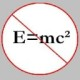You are currently browsing the tag archive for the ‘mathematical’ tag.

I thought I would share with our chess-loving readers the following interesting (and somewhat well-known) mathematical chess paradox , apparently proving that$64=65$, and the accompanying explanation offered by Prof. Christian Hesse, University of Stuttgart (Germany).  It shows a curious connection between the well-known Cassini’s identity (related to Fibonacci numbers) and the$8 \times 8$ chessboard ($8$ being a Fibonacci number!). The connection can be exploited further to come up with similar paradoxes wherein any$F_n \times F_n$ -square can always be “rerranged” to form a$F_{n-1} \times F_{n+1}$ -rectangle such that the difference between their areas is either$+1$ or$-1$. Of course, for the curious reader there are plenty of such dissection problems listed in Prof David Eppstein’s Dissection page.

This one, by Dr. Titu Andreescu (of USAMO fame), is elementary in the sense that the solution to the problem doesn’t require anything more than arguments involving parity and congruences. I have the solution with me but I won’t post it on my blog until Jan 19, 2008, which is when the deadline for submission is. By the way, the problem (in the senior section) is from the$6^{th}$ issue of Mathematical Reflections, 2007.

Problem: Find the least odd positive integer$n$ such that for each prime$\displaystyle p, \, \frac{n^2-1}{4} + np^4 + p^8$ is divisible by at least four (distinct) primes.

### Blog Stats

• 371,445 hitsStrong equidistribut… on Platonic Solids and Euler…m.s.mohamed ansari on The 54th Carnival of Math… Convictions ·… on Continued fraction for eWayne J. Mann on Solution to POW-12: A graph co…erneststephen on The 54th Carnival of Math…anhtraisg on p^q + q^p is primeprof dr drd horia or… on My first postprof drd horia orasa… on My first postprof dr mircea orasa… on Inequality with lognotedscholar on Self-referential Paradoxes, In…prof dr mircea orasa… on Inequality with logprof dr mircea orasa… on Inequality with logprof dr mircea orasa… on 2010 in reviewkenji on Basic category theory, Iprof dr mircea orasa… on Solution to POW-10: Another ha…